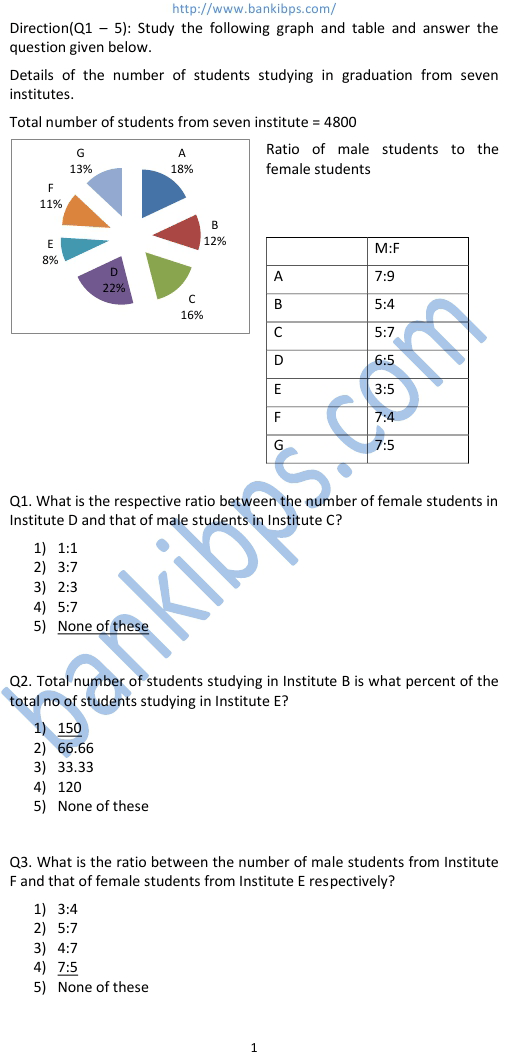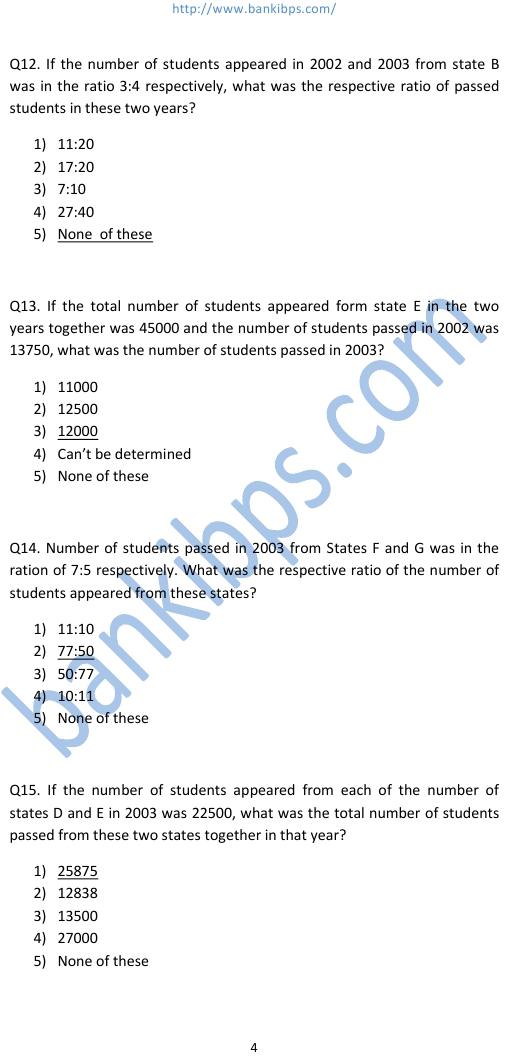# Data Interpretation Bank Exam

Q13. If the total number of students appeared form state E in the two years together was 45000 and the number data interpretation bank exam of students passed in 2002 was 13750, what was the number of students passed in 2003? 1) 11000 2) 12500 3) 12000 4) Can’t be determined 5) None of these Q14. Number of students passed in 2003 from States F and G was in the ration of 7:5 respectively. What was the respective ratio of the number of students appeared from these states? 1) 11:10 2) 77:50 3) 50:77 4) 10:11 5) None of these Q15. If the number of students appeared from each of the number of states D and E in 2003 was 22500, what was the total number of students passed from these two states together in that year? 1) 25875 2) 12838 3) 13500 4) 27000 5) None of these
Practice Exercise - 242 [ Data Interpretation ]## data interpretation bank exam

### test of data analysis and interpretation for bank exams

#### data analysis and interpretation for bank po exam

##### data analysis and interpretation questions for bank po exam
###### book for data interpretation
test of data analysis and interpretation for bank exams. data interpretation for bank exams. data analysis and interpretation for bank po exam. data analysis and interpretation for bank po. data analysis and interpretation questions for bank exams. data interpretation for bank po. data interpretation questions for bank po. data interpretation questions with solutions for bank exams. bank po data interpretation questions. data analysis and interpretation questions for bank po exam. data interpretation questions with solutions for bank po. book for data interpretation.